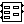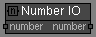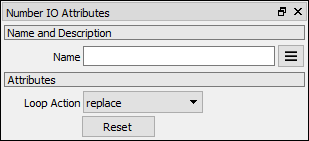# Number IO node

Left Toolbar >Project Nodes >Number IO

The Number IO node defines an input or output of NUMBER node value type in Custom nodes.

## Procedure

### To add a number input or output to a custom node:

1. On the left toolbar choose Project Nodes> Number IO2. Drag the Number IO icon into the Node Graph.3. Connect the input: number.

4. Connect the Output: number.

## Inputs

number

The number value that will be received by the custom node's node graph as input. Number IO nodes that have connected outputs are used by the Node Graph node as inputs in the custom node.

## Outputs

number

The number value that will be returned by the custom node's node graph as output Number IO nodes that have no connected outputs are used by the Node Graph node as outputs in the custom node.

## Attributes PanelLoop Action The type of action applied on this number node value after each loop:

replace: Replaces the previous value with the new value. summation: Adds the new value to the previous values. average: Adds the new value to the previous values and on the final loop divides the final value by the number of iterations.

Project Nodes

Main Page